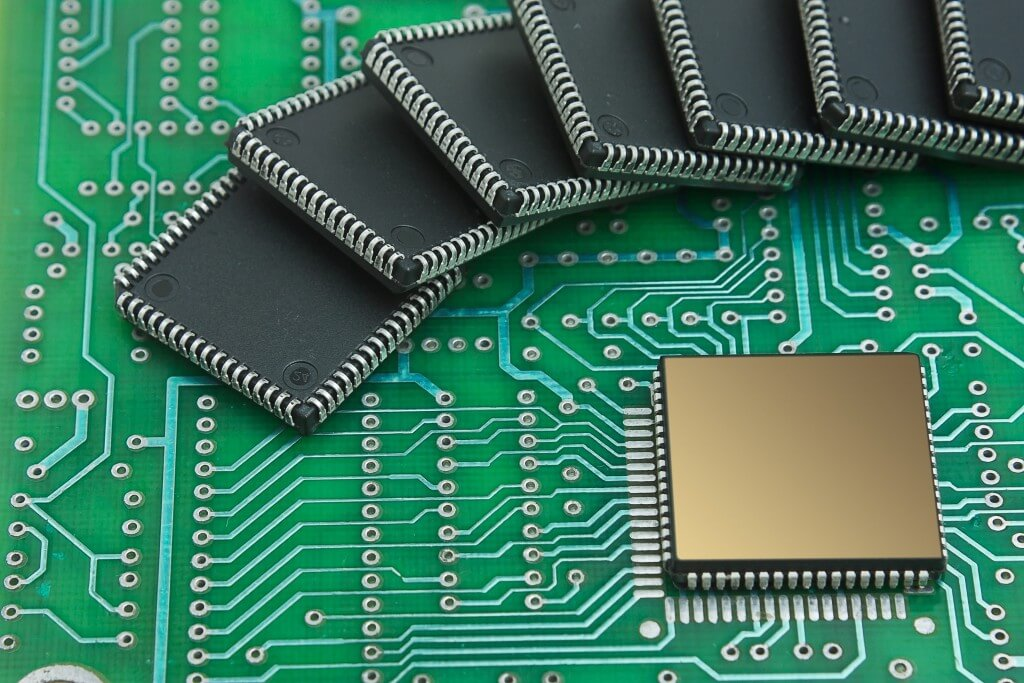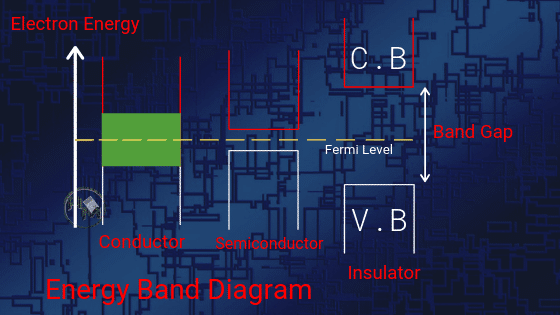Semiconductors are the backbone of electronics components. They are used more than conductors and find applications in every circuit. Be it a most commonly used multimeter or a device using rectifiers, transistors, voltage regulators, or anything in its circuit.

You also have heard about them in physics and chemistry in your academics.  But you don’t know what are they actually? How are these materials defined and what are their properties and uses? Don’t be sad. Below we are giving every basic detail of these materials that too in easy to get language. Let’s start our chapter now.

Before knowing about semiconductors, we need to have knowledge about conductors and insulators. So we will define them first.

Mainly there are 2 types of materials in electronics; conducting materials, and non-conducting materials.

1. Conductive materials are good carriers of electricity. They have ideally zero resistance. That’s being said, they allow free flow of charges in the circuit. Some examples of conducting materials are copper, aluminum, iron, silver, etc. These are also called Conductors.
2. Non-Conducting materials are bad conductors of electricity. They have infinite resistance and don’t allow electricity to pass through them. In electrical terms, these are called inductors. Examples of inductors are Wood, rubber, etc. In layman term, any material that doesn’t pass electricity through it is an inductor.

## What is a Semiconductor: Definition

We have read about two types of materials above. But there is a 3rd type of material who is neither completely a conductor and neither an insulator. The electrical property of this material lies between the two discussed above.A semiconductor is defined as a material that has electrical properties between a conductor and an insulator. The variation in electrical property is attained by introducing impurity in the material. This added impurity is defined as “Doping”.

These are of 2 types; Intrinsic and Extrinsic. They have negative temperature coefficient because its resistivity decrease with the increase of temperature or vice versa.

Germanium, Silicon, Gallium Arsenide are the most used and famous semiconductor materials used in the manufacturing of different electronic components.

## Energy band Theory

A Danish Physicist, Neil Bohr gave Energy Band theory also known as Solid state Band theory; based on the atomic model of the materials. According to this theory, he proposed,

Energy Band Theory Definition: Every atom has a discrete amount of energy. When atoms come together to form a compound, their atoms orbital energies mix to form molecular orbital energies.

When molecular orbitals are formed then many of the energy levels start to completely degenerate into energy. These energy levels are known as bands of energy.

At absolute 0K temperature, it acts as an insulator, that means it conducts no current. While above 0K temperature, it acts as a conductor. In Solid State band theory, different energy bands are:

(i) Valence band: The valence band is described as the outermost electron orbital of an atom. Valence bands are those bands where all of the valence electrons reside and are involved in the highest molecular orbit. These are either fully filled or partially filled.

(ii) Conduction band: These bands are empty or partially filled where positive or negative charge carriers exist. Negative charge carriers are the electrons while the positive charge carriers are holes.

(iii) Forbidden gap: The gap between the valence band and the conduction band is known as the forbidden gap. For an electron to jump from valence band to conduction band, it has to be energized by heat or electricity. The amount of energy required defines the conductivity of the material.

## Energy Band Diagram ExplanationWhen we apply an electric field, due to excitation, electrons start moving from valence band to conduction band and constitute a current.

As shown in the above figure, Conductors don’t have any forbidden gap between the valence band and conduction band. As the conduction band and valence band in conductors are already overlapped.

In insulators, there is a large gap between the conduction band and the valence band. This gap of approx 15ev indicates that insulators cannot conduct any current.

Though in semiconductors, there is a small forbidden gap between valence and conduction band. This indicates that more electrons can move and constitutes the current. From this theory, it is clear that the conductivity of a semiconductor always lies between conductor and insulator.

## Types of Semiconductors:

In a semiconductor, current can be carried out by the flow of electrons or by the flow of positively charged holes in the electron structure of the material. Depending on this property, semiconductors are classified into two categories:

### (i) Intrinsic semiconductor:

An intrinsic semiconductor is made up of pure semiconductor material. This means it is the one where the number of holes is equal to the number of electrons in the conduction band. The main characteristic of this type of semiconductor is the Fermi level which lies somewhere in between the valence band and the conduction band.

### (ii) Extrinsic semiconductor:

Extrinsic semiconductors are those where a small amount of impurity has been added to the basic intrinsic material. The phenomenon of adding an impurity to the material is known as doping.

The dopant materials chosen for doping are deliberately chosen in such a manner that either they have 3 electrons in their valence band or they have just 5 electrons in their valence band. Therefore such dopants are known as Trivalent and pentavalent impurity.

On the basis of this extrinsic type is again divided into two:

#### (A) P-type:

In this type, we add trivalent impurities such as boron, aluminum, gallium, etc to an intrinsic semiconductor.  This process of adding trivalent impurity creates deficiencies of valence electrons called holes.

Points to remember about P-type semiconductor are:

• It has a large number of holes.
• The acceptors are negatively charged.
• A small number of electrons in relation to the number of holes.
• Doping creates negatively charged acceptors and positively charged holes.

#### (B) N-type:

To create n-type, we add pentavalent impurities such as antimony, phosphorus, and arsenic. These dopants contribute free electrons, greatly increasing the conductivity of the intrinsic semiconductor.

Points to remember about n-type semiconductor are:

• It has a large number of free electrons.
• Donors are positively charged.
• A small number of holes in relation to the number of electrons.
• Doping gives positively charged donors and negatively charged free electrons.

## Applications of Semiconductor

Semiconductor devices are the key element of all electronic circuits. In every commercial product, they are used. From the family car to the pocket calculator, applications are:

• Voltage regulator circuits
• Rectifiers which are used in Dc power supplies
• Solid state devices, computers and data processing systems